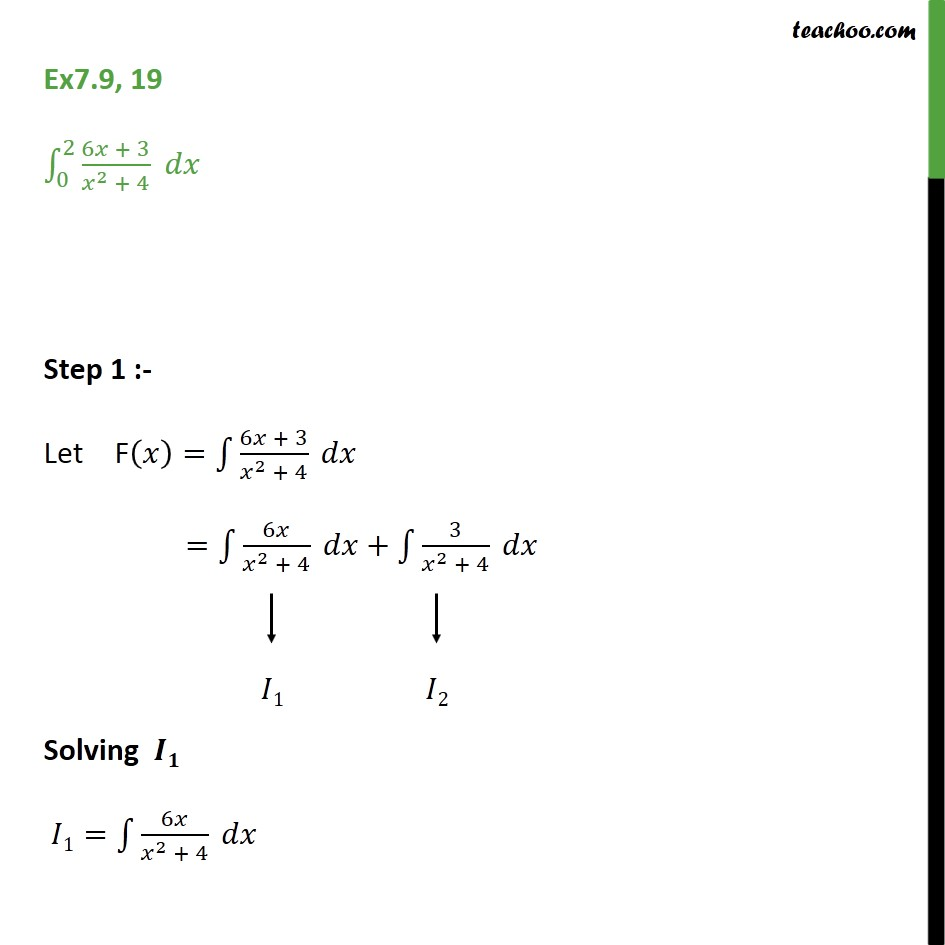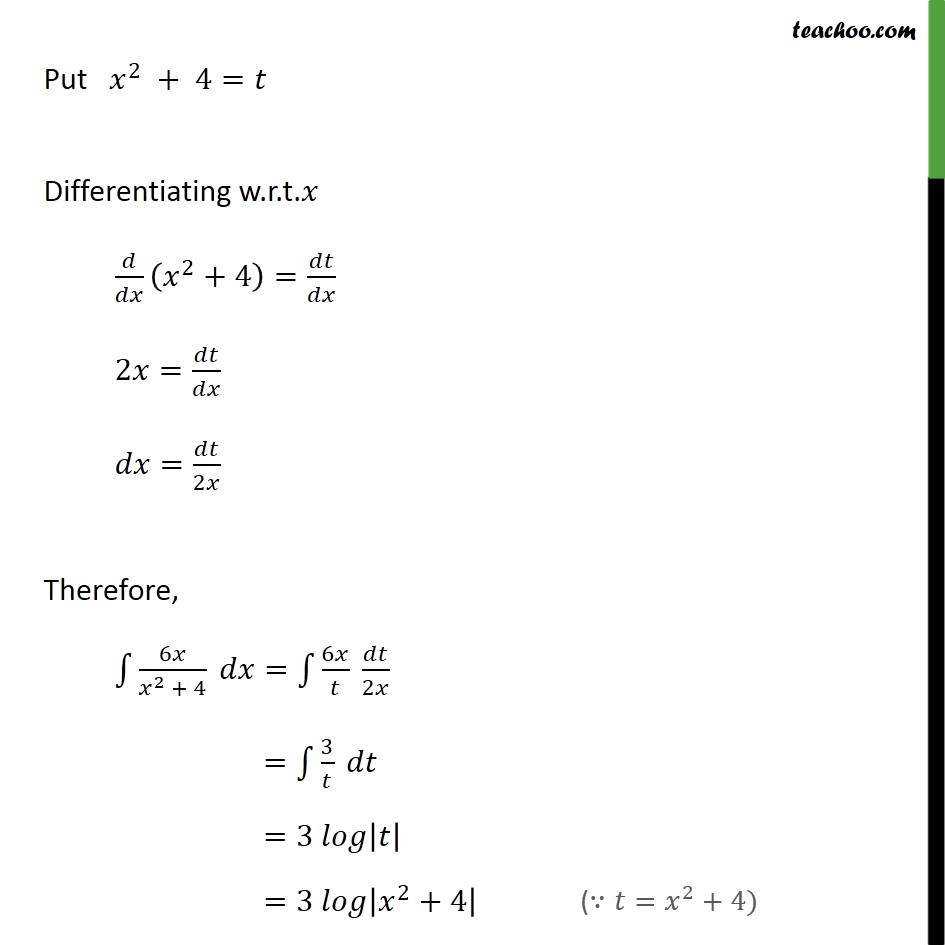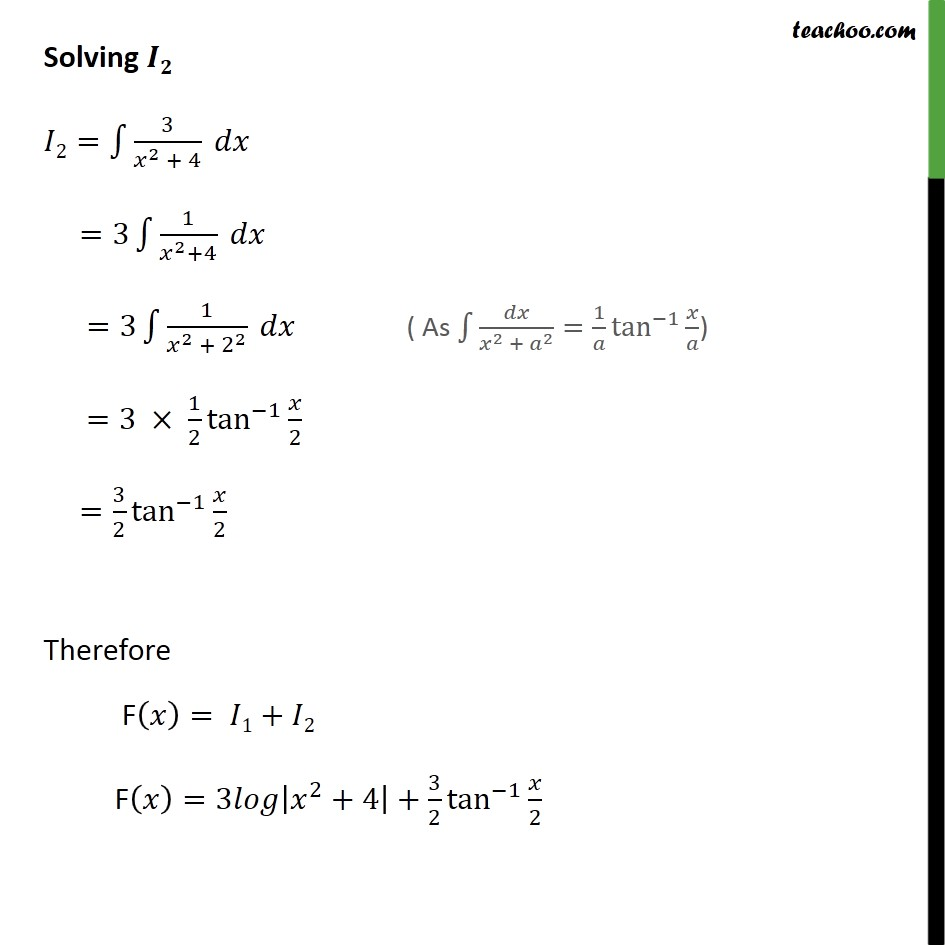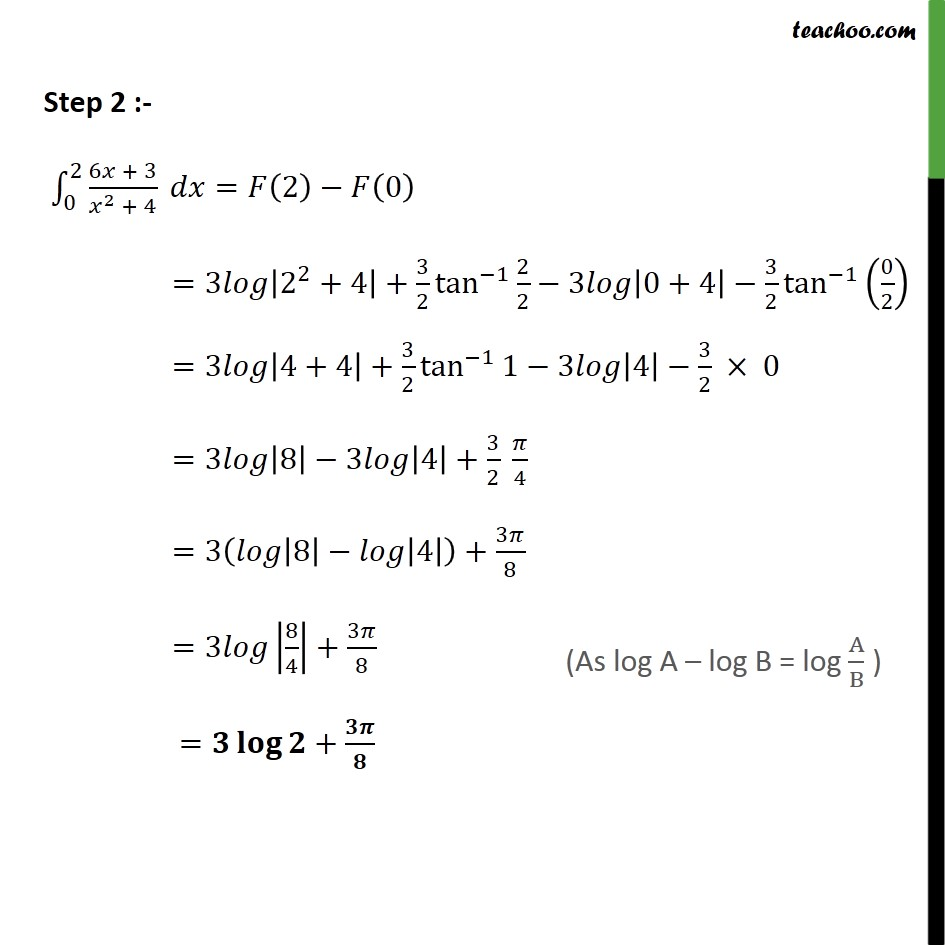1. Chapter 7 Class 12 Integrals
2. Concept wise
3. Definite Integration - By Formulae

Transcript

Ex7.9, 19 0 2 6 + 3 2 + 4 Step 1 :- Let F = 6 + 3 2 + 4 = 6 2 + 4 + 3 2 + 4 Solving 1= 6 2 + 4 Put 2 + 4= Differentiating w.r.t. 2 +4 = 2 = = 2 Therefore, 6 2 + 4 = 6 2 = 3 =3 =3 2 +4 Solving 2= 3 2 + 4 =3 1 2 +4 =3 1 2 + 2 2 =3 1 2 tan 1 2 = 3 2 tan 1 2 Therefore F = 1+ 2 F =3 2 +4 + 3 2 tan 1 2 Step 2 :- 0 2 6 + 3 2 + 4 = 2 0 =3 2 2 +4 + 3 2 tan 1 2 2 3 0+4 3 2 tan 1 0 2 =3 4+4 + 3 2 tan 1 1 3 4 3 2 0 =3 8 3 4 + 3 2 4 =3 8 4 + 3 8 =3 8 4 + 3 8 = +

Definite Integration - By Formulae